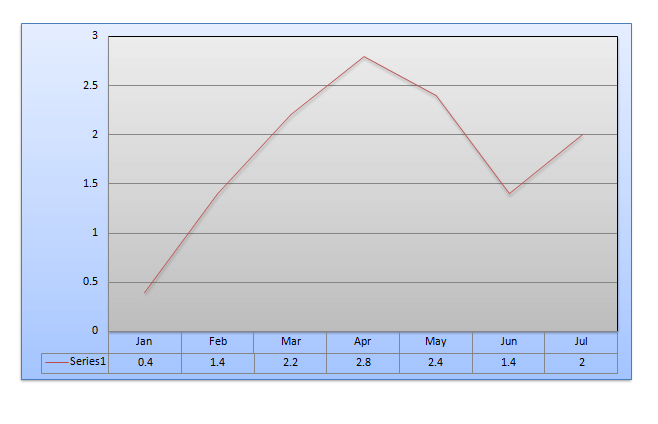## Wednesday, 22 March 2017

### DI QUIZ:BASED ON NEW PATTERN

Directions (Q. Nos. 1-4) Refer to the following line graph which represents the rainfall in- inches during the months of January through July in a particular year in a certain cityIn each of these questions, two quantities are given, one in Column A and one in Column B. Compare the two quantities.
(a) If the two quantities are equal
(b) If the quantity in column B is greater
(c) If the quantity in column A is greater
(d) If the relationship cannot be determined from the information given

Question 1.
Column A                                           Column B
The ratio of rainfall                                 2 : 3
in the months of
February and July
Question 2.
Column A                                            Column B
The average monthly                            1.9 inches
Rainfall recorded for the
7 months
Question 3.
Column A                                            Column B
25/2% of all the rain                          The amount of rainfall during the month of February
that fell during the 7
months period
Question 4.
Column A                                            Column B
The ratio between the                          The ratio between the rainfall in April and February
rainfall in May and January

Total rainfall in 7 months= 12.6= $12\frac{1}{2}$ =$\frac{25×12.6}{200}=$ 1.575 inch The amount of rainfall during the month of February = 1.4 inch The value of the column A is more. Therefore the correct option is (c).